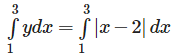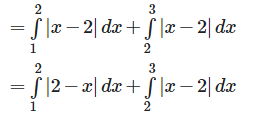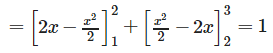# Test: Application Of Integrals (CBSE Level) - 1

## 25 Questions MCQ Test Mathematics (Maths) Class 12 | Test: Application Of Integrals (CBSE Level) - 1

Description
This mock test of Test: Application Of Integrals (CBSE Level) - 1 for JEE helps you for every JEE entrance exam. This contains 25 Multiple Choice Questions for JEE Test: Application Of Integrals (CBSE Level) - 1 (mcq) to study with solutions a complete question bank. The solved questions answers in this Test: Application Of Integrals (CBSE Level) - 1 quiz give you a good mix of easy questions and tough questions. JEE students definitely take this Test: Application Of Integrals (CBSE Level) - 1 exercise for a better result in the exam. You can find other Test: Application Of Integrals (CBSE Level) - 1 extra questions, long questions & short questions for JEE on EduRev as well by searching above.
QUESTION: 1

###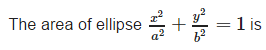Solution:

Area of standard ellipse is given by :πab.

QUESTION: 2

### The area bounded by the curve y = 4x - x2 and the x- axis is equal to

Solution:

For x - axis, y = 0,

Therefore, 4x - x2 = 0

Therefore, x = 0 or x = 4

Required area :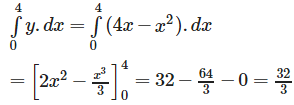QUESTION: 3

### The area of the smaller segment cut off from the circle x2+y2 = 9by x = 1 is

Solution: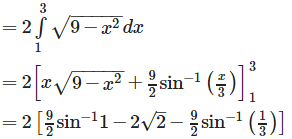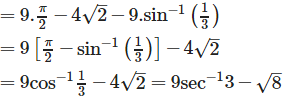QUESTION: 4

For which of the following values of m , is the area of the region bounded by the curve y = x - x2 and the line y = mx equal to 9/2 ?

Solution: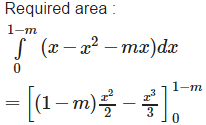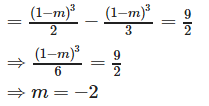QUESTION: 5

The area of the region bounded by the parabola (y−2)2 = x−1,the tangent to yhe parabola at the point (2 , 3) and the x – axis is equal to

Solution: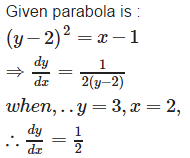Therefore, tangent at (2, 3) is y – 3 = ½ (x – 2). i.e. x – 2y +4 = 0 . therefore required area is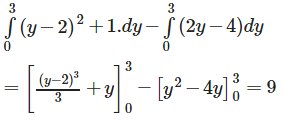QUESTION: 6

The area of the loop between the curve y = a sin x and the x – axis and x = 0 , x = π. is

Solution: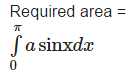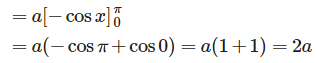QUESTION: 7

The area bounded by the curve y2 = x,line y = 4 and y – axis is equal to

Solution: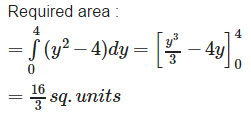QUESTION: 8

The area enclosed by the curve xy2 = a2(a − x) and the y – axis is

Solution: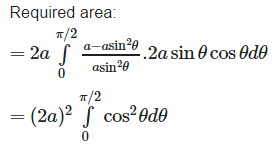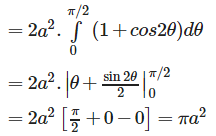QUESTION: 9

The area bounded by the curve y = x[x], the x – axis and the ordinates x = 1 and x = -1 is given by

Solution: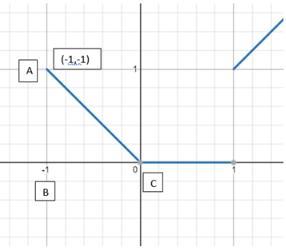Graph of y = x [x]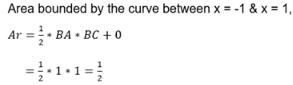QUESTION: 10

The area bounded by the curves y = cos x and y = sin x between the ordinates x = 0 and x = π/2 is equal to

Solution: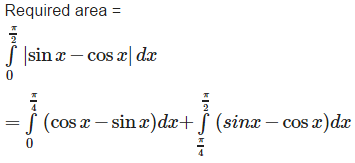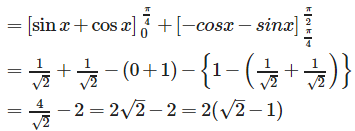QUESTION: 11

The area enclosed between the curves y = x3 ,x- axis and two ordinates x = 1 to x = 2 is [in square units]

Solution: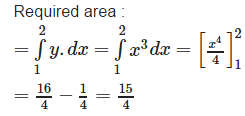QUESTION: 12

The area bounded by y = log x , the x – axis and the ordinates x = 1 and x = 2 is

Solution: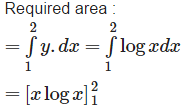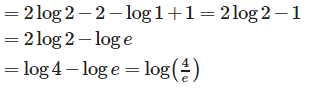QUESTION: 13

The area bounded by the curves y2 = x3 and |y| = 2x is given by

Solution:

Both  y2 = x3 and |y| = 2x are symmetric about y – axis and on solving them we get :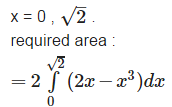QUESTION: 14

The area bounded by the curve y = 2x - x2 and the line x + y = 0 is

Solution:

The equation y = 2x − x2 i.e. y – 1 = - (x - 1)2 represents a downward parabola with vertex at (1, 1) which meets x – axis where y = 0 .i .e . where x = 0 , 2. Also , the line y = - x meets this parabola where – x = 2x − x2 i.e. where x = 0 , 3.
Therefore , required area is :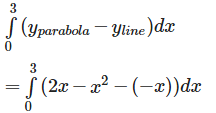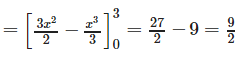QUESTION: 15

The area bounded by the parabola y = x2 and the line y = x is

Solution: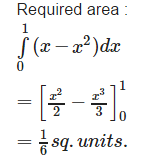QUESTION: 16

Area of the region bounded by the curves y = ex, x = a , x = b and the x- axis is given by

Solution: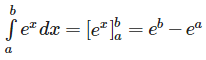QUESTION: 17

The area bounded by the curves y2 = 4x and y = x is equal to

Solution:

The two curves y2 = 4x and y = x meet where x2 = 4x i.e ..where x = 0 or x = 4 . Moreover , the parabola lies above the line y = x between x = 0 and x = 4 . Hence , the required are is :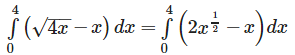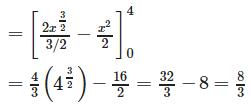QUESTION: 18

The area bounded by the curves y = √x, 2y+3 = x and the x- axis in the first quadrant is

Solution:

To find area the curves y = √x and x = 2y + 3 and x – axis in the first quadrant., We have ; y2−− 2y −− 3 = 0 (y – 3) (y + 1) = 0 . y = 3 , - 1 . In first quadrant , y = 3 and x = 9.
Therefore , required area is ;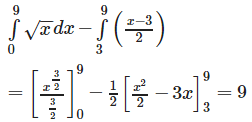QUESTION: 19

Let y be the function which passes through (1 , 2) having slope (2x + 1) . The area bounded between the curve and the x – axis is

Solution:

Given slope of the curve is 2x + 1.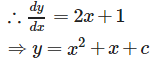Also , it passes through (1, 2).
∴ 2 = 1+1+c ⇒ c = 0
Equation of curve is : y = x2 + x. Therefore , points of intersection of y = x (x +1) and the x – axis are x = 0 , x = - 1.
Required area :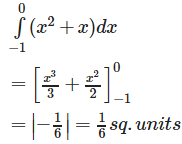QUESTION: 20

AOB is the positive quadrant of the ellipse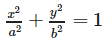in which OA = a and OB = b . The area between the arc AB and chord AB of the ellipse is

Solution:

Required area :
= (1/4) area of ellipse – area of right angled triangle AOB.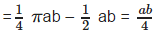(π - 2)

QUESTION: 21

The area common to the circle x2+y2 = 16 and the parabola y2 = 6x is

Solution: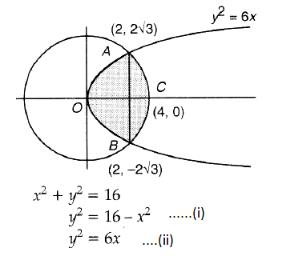Solving eqns. (i) and (ii), we get points of intersection (2, 2√3) and (2, -2√3)

Substituting these values of x in eq. (ii). Since both curves are symmetrical about r-axis.

Hence the required area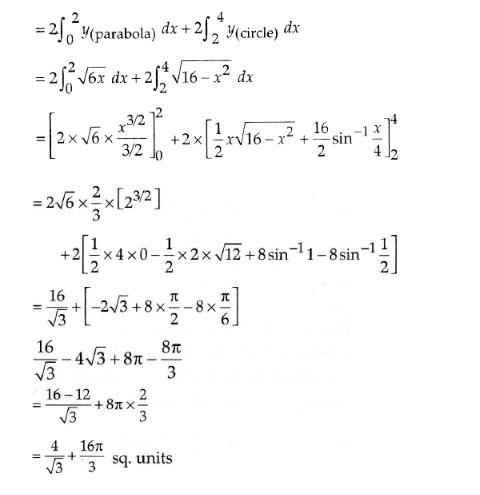QUESTION: 22

The area included between the curves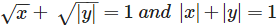is equal to

Solution:

Required area :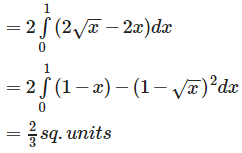QUESTION: 23

The area bounded by the curves y2 = 20x and x2 = 16y is equal to

Solution:

Eliminating y, we get :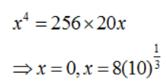Required area: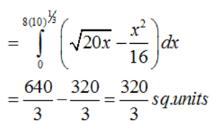QUESTION: 24

The area bounded by the curves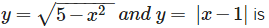Solution:

Required area :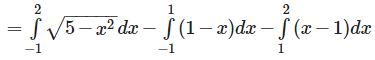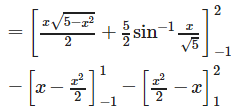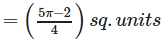QUESTION: 25

The area of the region bounded by the curves y = |x−2|, x = 1 , x = 3 and the x – axis is

Solution: